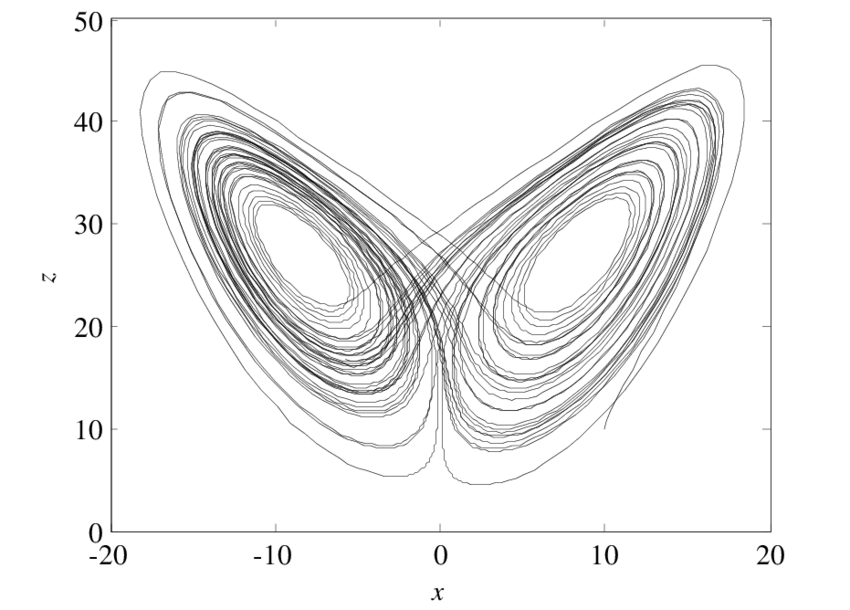# Nonlinear DynamicsNonlinear dynamics is a branch of mathematics and physics that deals with the study of systems that exhibit complex behavior. The main difference between linear and nonlinear systems is that linear systems can be described by linear equations, while nonlinear systems are described by nonlinear equations. Linear equations are equations that can be written in the form of ax + b = 0, where a and b are constants, while nonlinear equations are equations that cannot be written in that form.
Linear systems are relatively easy to analyze and predict, while nonlinear systems are much more complex. Linear systems exhibit a behavior that is predictable and repeatable, while nonlinear systems exhibit a behavior that is often chaotic and unpredictable. Because of this, nonlinear systems are much harder to model mathematically. In order to understand nonlinear systems, we need to use more advanced mathematical tools such as multivariable differential equations, and chaos theory.
Linear algebra is a branch of mathematics that deals with the study of linear equations and their solutions. It is used to analyze and understand the behavior of linear systems. Linear algebra provides a powerful toolset for solving systems of linear equations and understanding the properties of linear systems. Events are described in simple terms and considered in a matrix with how the variables relate to each other. It is not, however, well suited for understanding the behavior of nonlinear systems.
Nonlinear dynamics, on the other hand, is the study of nonlinear systems and the behavior of these systems. It uses a combination of linear algebra, differential equations, and chaos theory to analyze and understand the behavior of nonlinear systems. Nonlinear dynamics provides a powerful toolset for understanding the behavior of nonlinear systems, but it also has its limitations.